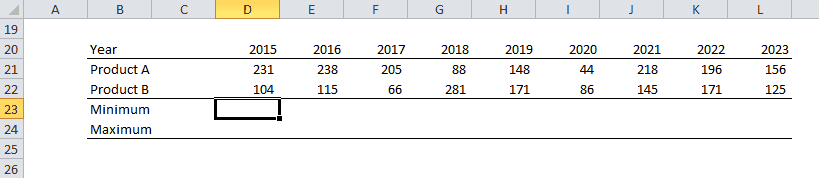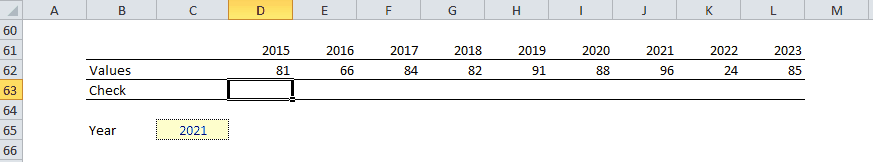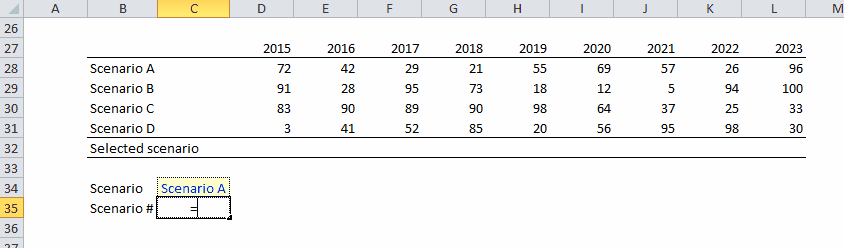top of page

Excel functions

## Syntax:=sum(Range)

Using Excel in a different language? Find the translations of the functions here.SUMIF

## Syntax:=sumif(Range of the condition, Condition, Range of the values)SUMPRODUCT

Multiplies corresponding array components and sums them

Syntax:=sumproduct(array #1 , array #2, etc.)

The array must have exactly the same size (number of cells)MIN/MAX

Returns the maximum (minimum) value from a range

## Syntax:=min(range) or min(cell #1 , cell #2, etc.)IF

Returns a value if a condition is True and another value if it is False

Syntax:=if(condition ,  value if condition is True , value if condition is false)

In the example below, the if function return the value if the year is inferior to 2021 and na else.IFERROR

Returns a specific value if the tested formula returns an error, else it returns the formula result

Syntax:=iferror(formula ,  value if the result is an error)MATCH

## Syntax:=match(Value to look for, Range, Type (always put 0))CHOOSE

## It is very powerful when used with match as it allows to switch very quickly from one scenario to the otherINDEX

## It is very powerful when used with matchOFFSET

## It can be used like INDEX to return a signe value:But it can also be used to create a dynamic range, i.e. a range that does not have a fixed size:Using Excel in a different language? Find the translations of the functions here.

bottom of page